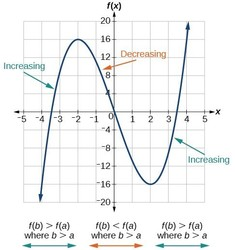# Increasing and Decreasing functionIncreasing Function:
A function y=f(x) is said to be increasing function in the interval (a, b) if for every x1x2 ∈ (a, b)
x2 x﻿﻿=> f(x﻿﻿) > f(x1)
Slope of tangent at any point of such curve is always positive.
i.e., f'(x)>0GRAPH OF INCREASING FUNCTION

Decreasing Function:
A function y=f(x) is said to be decreasing function in the interval (a, b) if for every x1, x2 ∈ (a,b)
x2 > x1 ﻿﻿=> f(x﻿﻿) < f(x1)|
Slope of tangent at any point of such curve is always negative.
i.e., f'(x)<0GRAPH OF DECREASING FUNCTION

﻿﻿